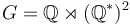# Endomorph-dominating not implies automorph-conjugate

This article gives the statement and possibly, proof, of a non-implication relation between two subgroup properties. That is, it states that every subgroup satisfying the first subgroup property (i.e., endomorph-dominating subgroup) need not satisfy the second subgroup property (i.e., automorph-conjugate subgroup)
View a complete list of subgroup property non-implications | View a complete list of subgroup property implications
It is possible to have a group$G$ and a subgroup$H$ such that both the following hold:
•$H$ is an endomorph-dominating subgroup of$G$: For every endomorphism$\sigma$ of$G$, there exists$g \in G$ such that$\sigma(H) \le gHg^{-1}$.
•$H$ is not an automorph-conjugate subgroup of$G$: There exists an automorphism$\sigma$ of$G$ such that$H$ and$\sigma(H)$ are not conjugate subgroups.
We take$G = \mathbb{Q} \rtimes (\mathbb{Q}^*)^2$ and$H$ as a copy of$\mathbb{Z}$ inside the base$\mathbb{Q}$.